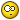# Why getvar is not working?## Recommended PostsCan someone help me on why this code is throwing nil?

```(command "_area")

(while (> (getvar "cmdactive") 0) (command pause))

(setq e (getvar "_area"))```

e has nil value...

##### Share on other sitesTry:

```(command "_.area")
(while (> (getvar "cmdactive") 0) (command pause))
(setq e (getvar "area"))```

##### Share on other sitesI don't believe that getvar honors the "_"

Try :

```(getvar "AREA")
```

-David

##### Share on other sites(Nop Nop)

Neither has worked !

##### Share on other sites(getvar "AREA") has worked.

Since when area should be written in capitals ????##### Share on other sitesok, now why this is giving a "bad function"?

``` (if (= fuel "a") ((setq kw (getreal))
(setq p (* 6 kw))
(setq a (* 0.25 k)))
((setq p (* 0.5 e))
(setq a (* 0.25 k)))

)```

##### Share on other sitesNeither has worked !

You have certainly done something wrong.

ok, now why this is giving a "bad function"?

```(if (= fuel "a")
(progn
(setq kw (getreal))
(setq p (* 6 kw))
(setq a (* 0.25 k))
)
(progn
(setq p (* 0.5 e))
(setq a (* 0.25 k))
)
)
```

##### Share on other sites```(if (= fuel "a")
(setq kw (getreal "\nHow many Km?")
p  (* 6 kw)
a  (* 0.25 k)
);; setq
(setq kw (getreal "\nHow many Km?")
p  (* 0.5 e)
a  (* 0.25 k)
);; setq
);; if
;;or

(if (= fuel "a")
(progn
(setq kw (getreal "\nHow many Km?"))
(setq p (* 6 kw))
(setq a (* 0.25 k))
);; progn
(progn
(setq kw (getreal "\nHow many Km?"))
(setq p (* 0.5 e))
(setq a (* 0.25 k))
);; progn
);; if
```

##### Share on other sitesok, now why this is giving a "bad function"?

``` (if (= fuel "a") ((setq kw (getreal))
(setq p (* 6 kw))
(setq a (* 0.25 k)))
((setq p (* 0.5 e))
(setq a (* 0.25 k)))

)```

Use progn

```(if (= fuel "a")
(progn
(setq kw (getreal))
(setq p (* 6 kw))
(setq a (* 0.25 k))
)
(progn
(setq p (* 0.5 e))
(setq a (* 0.25 k))
)
)
```

Or combine the grouped setqs

```(if (= fuel "a")
(setq kw (getreal)          p (* 6 kw)          a (* 0.25 k)  )
(setq p (* 0.5 e)          a (* 0.25 k) )
)```

## Join the conversation

You can post now and register later. If you have an account, sign in now to post with your account.
Note: Your post will require moderator approval before it will be visible.Reply to this topic...

×   Pasted as rich text.   Restore formatting

Only 75 emoji are allowed.